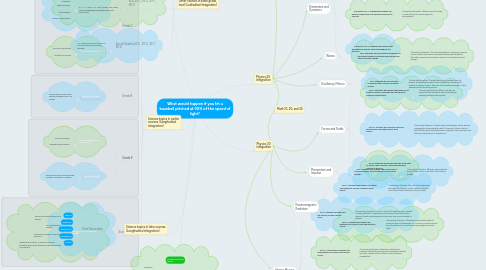What would happen if you hit a baseball pitched at 90% of the speed of light?Neil Whaley
Get Started. It's FreeWhat would happen if you hit a baseball pitched at 90% of the speed of light?1. Science topics in earlier courses (Longitudinal integration)

1.1.1. Heat and Temperature

1.1.1.1. Particle model of matter

1.1.1.2. Thermal energy

1.1.1.3. Heat transfer

1.1.1.4. Energy conservation

1.1.2. Structures and Forces

1.1.2.1. Forces on structures

1.1.2.2. Direction of forces

1.2.1. Mechanical Systems

1.2.1.1. Transmission of force and energy between parts of a system

1.3.1. Electrical Principles and Technologies

1.3.1.1. Forms of energy

1.3.1.2. Energy transformation

1.3.2. Space Exploration

1.3.2.1. Reference frames for describing position and motion in space

1.4. Science 10

1.4.1. Energy Flow in Technological Systems

1.4.1.1. Forms and interconversions of energy

1.4.1.2. One-dimensional motion

1.4.1.3. Mechanical energy conversions and work

1.4.1.4. Design and function of devices involving potential and kinetic energy and thermal energy conversions

2. Science topics in later courses (Longitudinal integration)

2.1. Post-Secondary

2.1.1. Physics

2.1.2. Chemistry

2.1.3. Mathematics

2.1.4. Engineering

2.1.5. Villainy

3.2.1. baseballs

3.2.2. cars

3.2.3. toboggans

3.3. Nuclear Energy

3.3.1. fission

3.3.2. fusion

3.3.3. environmental issues

3.4. Magnets & Magnetic Fields

3.4.1. electronics

3.4.2. fMRI

3.5.1. properties of light

3.5.2. microwaves

3.5.3. cell phones & communication

7. Momentum and Impulse

7.1. GLO: Students will explain how momentum is conserved when objects interact in an isolated system.

7.1.1. Connecting Question: What do you predict to be the shape of the crater left at the old ball game?

8. Forces and Fields

8.1. GLO 1: Students will explain the behaviour of electric charges, using the laws that govern electrical interactions.

8.1.1. Connecting Question: What is the the net magnetic field caused by the ball of plasma travelling towards home plate?

8.2. GLO 2: Students will describe electrical phenomena, using the electric field theory.

8.2.1. Connecting Question: Predict what would happen to the plasma components surrounding the ball if it were put into an electric field? What effect would the earth's magnetic field have upon the the ions and electrons in the plasma?

9.1. GLO 1: Students will explain the nature and behaviour of EMR, using the wave model.

9.1.1. Connecting Question: Describe the relationship between the intensity of the radiation emitted from the ball and the distance from the ball.

9.2. GLO 2: Students will explain the photoelectric effect, using the quantum model.

9.2.1. Connecting Question: The plasma surrounding the ball emits photons of wavelength of X, does your aluminum bat emit any electrons in response to being exposed to this light?

10. Atomic Physics

10.1. GLO 1:Students will describe the electrical nature of the atom

10.1.1. Connecting Question: The air in front of the ball becomes heated through adiabatic compression to the point where it becomes plasma. Explain what happens to atoms as they are converted to plasma.

10.2. GLO 2: Students will describe the quantization of energy in atoms and nuclei.

10.2.1. Connecting Question: Determine whether the photons emitted from the plasma created by the ball will have a photoelectric effect on the aluminum baseball bat.

10.3. GLO 3: Students will describe nuclear fission and fusion as powerful energy sources in nature.

10.3.1. Connecting Question: Describe what happens to the plasma in front of the ball as temperature and pressure reach sufficient values to sustain fusion.

11. Oscillatory Motion

11.1. GLO: Students will describe the conditions that produce oscillatory motion.

11.1.1. Connecting Question: The radiation that is emitted from the plasma surrounding the ball can be modelled as waves which exhibit oscillatory motion. Describe the wavelike properties of the quanta emitted from the plasma.

12. Waves

12.1. GLO: Students will describe the properties of mechanical waves and explain how mechanical waves transmit energy.

12.1.1. Connecting Question: The resulting explosion of energy causes a seismic shock wave that can be detected around the planet. Describe compression and shear waves. How do they transmit energy?

13. Circular Motion, Work, and Energy

13.1. GLO 1: Students will explain circular motion, using Newton’s laws of motion.

13.1.1. Connecting Question: As you swing the bat, describe the forces acting on it.

13.2. GLO 2: Students will explain that work is a transfer of energy and that conservation of energy in an isolated system is a fundamental physical concept.

13.2.1. Connecting Question: Calculate the kinetic energy of the ball (ignoring relativistic effects)

14. Kinematics and Dynamics

14.1. Kinematics GLO: Students will describe motion in terms of displacement, velocity, acceleration and time.

14.1.1. Connecting Question: How long will it take the ball to reach the home plate?

14.1.2. Connecting Question: The ball is redirected via an elastic collision to be travelling towards first base, what is it's displacement after X time?

14.2. Dynamics GLO 1: Students will explain the effects of balanced and unbalanced forces on velocity.

14.2.1. Connecting Question: What forces are acting on the ball as it moves through the atmosphere?

15. Other courses in same grade level (Latitudinal integration)

15.1. Math 20-1, 20-2, 30-1, 30-2

15.1.1. Many outcomes

15.2. ELA 20-1, 20-2, 30-1, 30-2

15.2.1. GLO 2: Students will listen, speak, read, write, view and represent to comprehend literature and other texts in oral, print, visual and multimedia forms, and respond personally, critically and creatively.

15.2.2. GLO 3: Students will listen, speak, read, write, view and represent to manage ideas and information.

15.3. Social Studies 20-1, 20-2, 30-1, 30-2

15.3.1. S.1: Student will develop skills of critical thinking and creative thinking